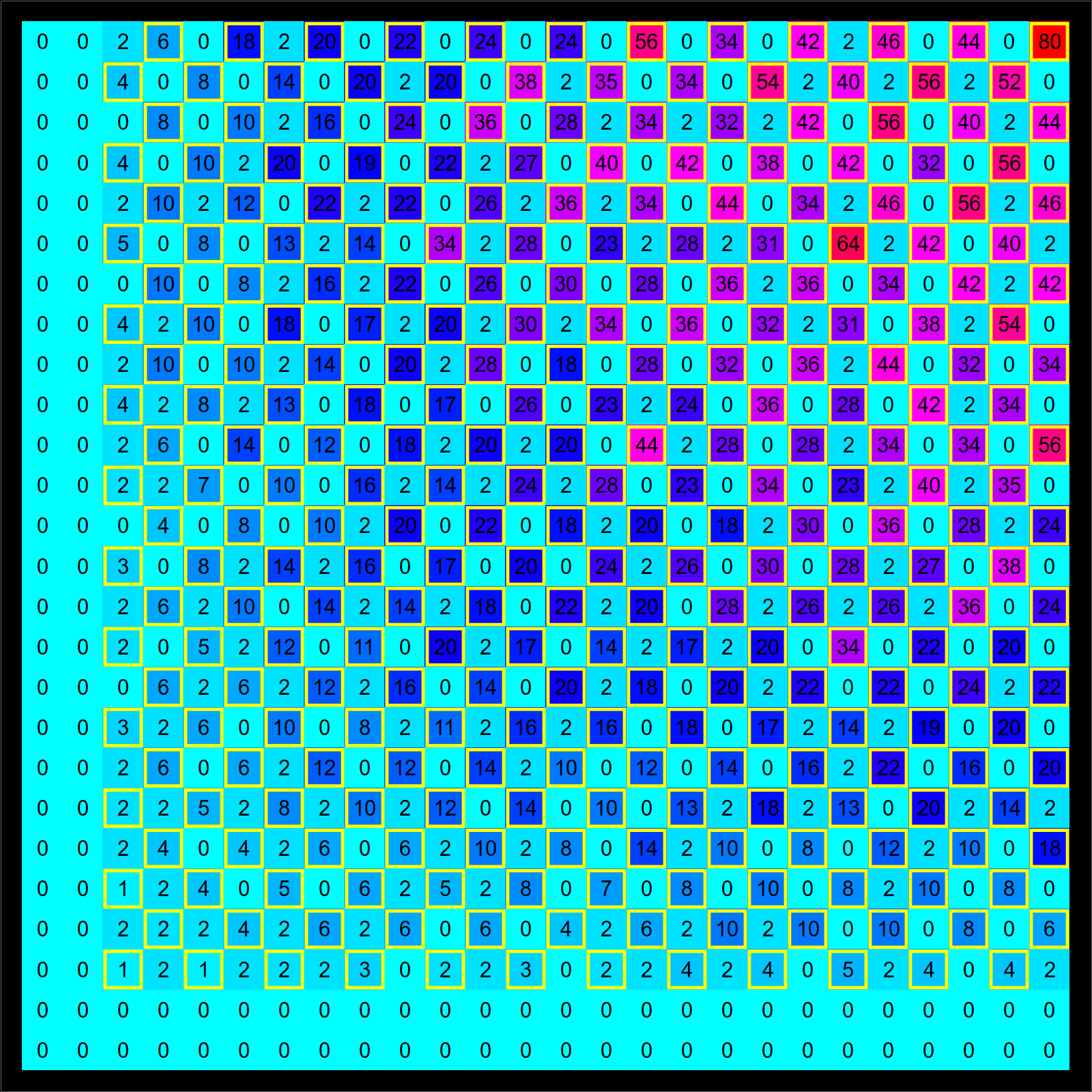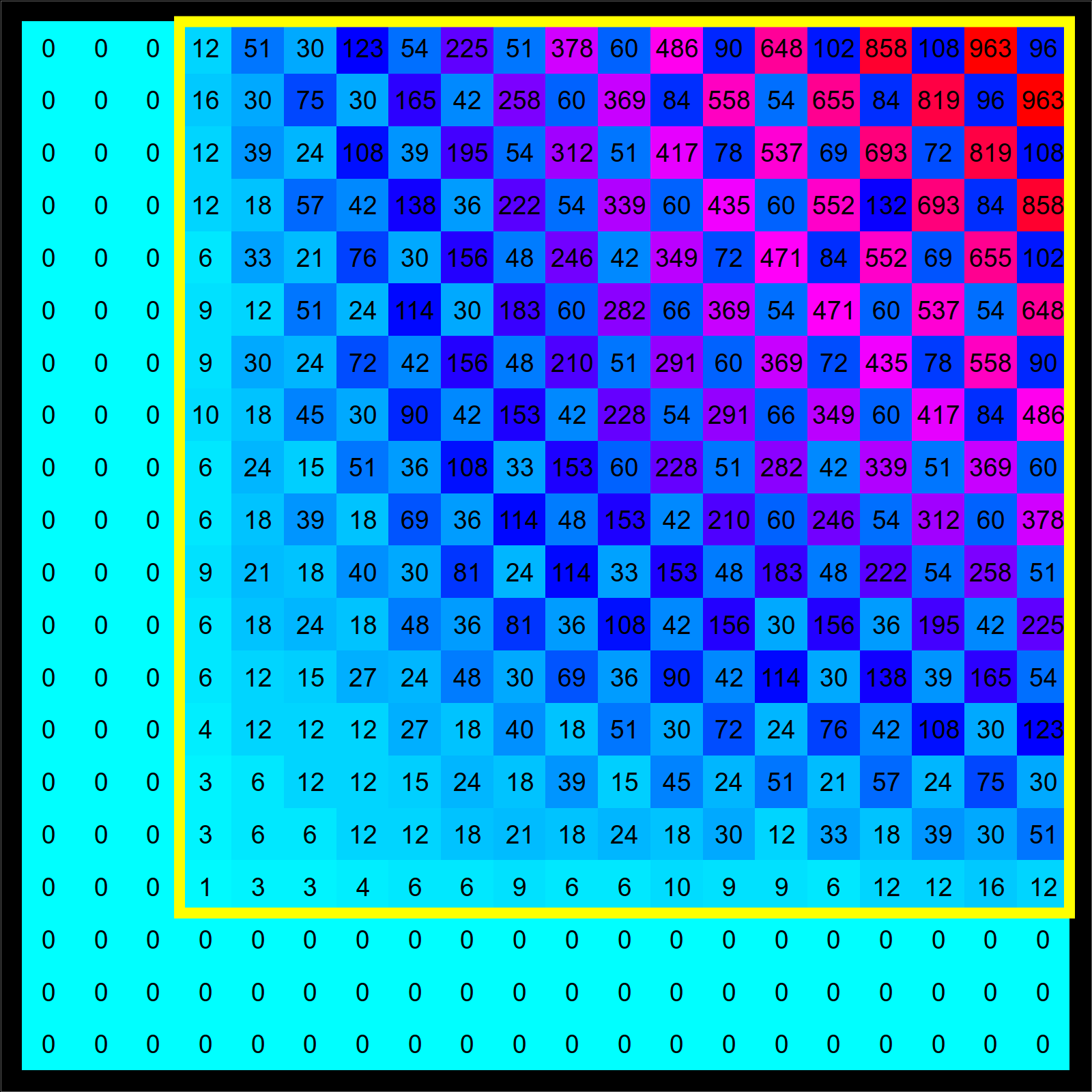# Goldbach for Gaussian Primes

I have been fascinated by the Goldbach problem since highschool: an episode. While writing an exam for Math21b (Spring 2016) which dealt with Gaussian primes, I began to experiment with these numbers. Of course also with Goldbach. A natural Gaussian Goldbach conjecture is that

 Every even Gaussian integer a+ib with a>1,b>1 is the sum of two Gaussian primes in the open first quadrant Q={ a+ib | a>0,b>0 }.

A Gaussian integer is an element in the ring Z[i]. It is even if it is a multiple of 1+i. Gaussian integers have a unique prime factorization modulo units U={1,i,-1,-i}. A Gaussian integer is prime if it can not be written as a product of two integers which both have smaller norm. The arithmetic norm of an integer a+ib is defined as a2 + b2. Gaussian primes must have prime norm or prime length. Indeed, there are two classes of Gaussian primes. The ones on the axes which are of the form u(4k+1), where u is a unit, or then primes a+ib with prime norm 4k+1 = a2 + b2. If A is the dihedral group generated by U and complex conjugation, then the orbifold C/A can best be visualized as a cone. The usual rational primes {2,3,5,7,11,...} are in 1-1 correspondence with the Gaussian primes on C/A.

This is not the first conjecture on Goldbach for Gaussian primes. There is one by Holben and Jordan from 1968 which assumes an angle condition. There is one by Rademacher 1925 and Mitsui from 1960 for general number fields. Both appear different.To Goldbach: if we look at integers of the form a+2i with even a, then the conjecture tells that we can write it as a sum of two primes p = n+i and q = m+i. Now, this especially means that n2 + 1 and m2 + 1 are both primes.

 The Gaussian Goldbach conjecture implies the Landau problem about the infinitude of primes of the form n2 + 1.

Since the Landau problem is widely believed to be hard, the Goldbach conjecture for Gaussian primes is not trivial. The conjecture is natural since it tells that if f(x,y) = suma + i b prime xa yb, then f(x,y)2 has positive derivatives dm/dxm dn/dxn f(x,y) for all even n+m, n>1,m>1 at the point (x,y)=(0,0). It is a calculus problem for a concrete function.
Here is the corresponding matrix for the ternary Gaussian Goldbach problem which asks whether

 TERNARY GAUSSIAN GOLDBACH: Every Gaussian integer with entry larger than 2 is the sum of three Gaussian primes in the open first quadrant.

The calculus reformulation is that f(x,y)3 has positive derivatives dm/dxm dn/dxn f(x,y) for all n > 2, and m > 2 at the point (x,y)=(0,0).